# apskmod

Amplitude phase shift keying (APSK) modulation

## Syntax

``y = apskmod(x,M,radii)``
``y = apskmod(x,M,radii,phaseoffset)``
``y = apskmod(___,Name,Value)``

## Description

example

````y = apskmod(x,M,radii)` performs APSK modulation on the input data, `x`, based on the specified number of constellation points per PSK ring, `M`, and the radius of each PSK ring, `radii`. For a description of APSK modulation, see Algorithms. Noteapskmod specifically applies to multiple ring PSK constellations. For a single ring PSK constellation, use `pskmod`. ```

example

````y = apskmod(x,M,radii,phaseoffset)` specifies an initial phase offset for each PSK ring of the APSK modulated signal.```

example

````y = apskmod(___,Name,Value)` specifies options using one or more name-value pair arguments using any of the previous syntaxes. For example, `'OutputDataType','double'` specifies the desired output data type as double. Specify name-value pair arguments after all other input arguments.```

## Examples

collapse all

Modulate data using APSK with an unequal number of constellation points on each circle.

Define vectors for modulation order and PSK ring radii. Generate data for constellation points.

```M = [4 8 20]; radii = [0.3 0.7 1.2]; modOrder = sum(M); x = 0:modOrder-1;```

Apply APSK modulation to the data.

`y = apskmod(x,M,radii);`

Plot the resulting constellation using a scatter plot.

`scatterplot(y)`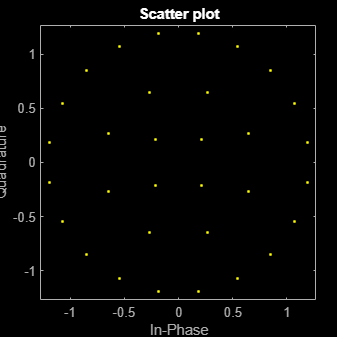Modulate a random data sequence using APSK with zero phase offset for the inner circle and pi/6 phase offset for the outer circle.

Define vectors for modulation order, PSK ring radii, and PSK ring phase offset. Generate random data.

```M = [8 8]; modOrder = sum(M); radii = [0.5 1]; phOff = [0 pi/6]; x = randi([0 modOrder-1],100,1);```

Apply APSK modulation to the data.

`y = apskmod(x,M,radii,phOff);`

Plot the resulting constellation using a scatter plot and observe the phase offset between the constellation circles.

`scatterplot(y)`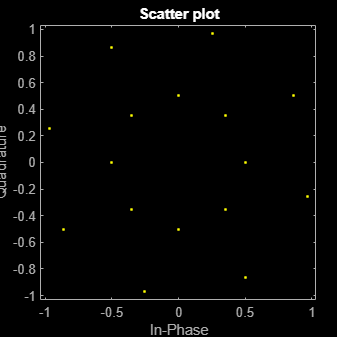Plot APSK constellations for gray and custom symbol mappings.

Define vectors for modulation order and PSK ring radii. Generate bit data for constellation points.

```M = [8 8]; modOrder = sum(M); radii = [0.5 1.5]; x = 0:modOrder-1;```

The `apskmod` function assumes the single channel binary input is left-msb aligned and specified column-wise. Reshape the bit matrix to express the single channel signal in a single column.

```xBit = de2bi(x,'left-msb'); xBit = xBit.';```

Apply APSK modulation to the data using the default phase offset. Since element values for `M` are equal and element values for phase offset are equal, the symbol mapping defaults to `'gray'`. Binary input is used to highlight the Gray nature of the constellation mapping. Plot the constellation.

`y = apskmod(xBit(:),M,radii,'PlotConstellation',true,'InputType','bit');`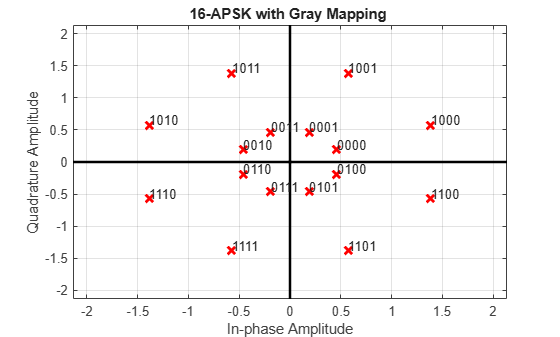Create a custom symbol mapping vector. This custom mapping happens to be another Gray mapping.

`cmap = [0;1;9;8;12;13;5;4;2;3;11;10;14;15;7;6];`

Apply APSK modulation with a custom symbol mapping. Plot the constellation. Binary input is used to highlight that the custom mapping defines different Gray symbol mapping.

`z = apskmod(xBit(:),M,radii,'SymbolMapping',cmap,'PlotConstellation',true,'InputType','bit');`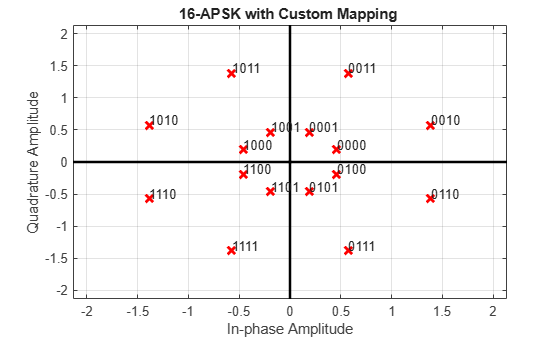Modulate a random bit sequence using APSK and output data type `single`. Pass the signal through a noisy channel and display the constellation diagram.

Define vectors for modulation order and PSK ring radii. Generate random binary data.

```M = [8 12 20 24]; radii = [0.8 1.2 2 2.5]; bitsPerSym = log2(sum(M)); x = randi([0 1],2000*bitsPerSym,1);```

Apply APSK modulation to the data and use a name-value pair to output as data type `single`.

`y = apskmod(x,M,radii,'InputType','bit','OutputDataType','single');`

Pass through an AWGN channel with a 25 dB SNR.

`yrec = awgn(y,25,'measured');`

Plot the received constellation as a scatter plot.

`scatterplot(yrec)`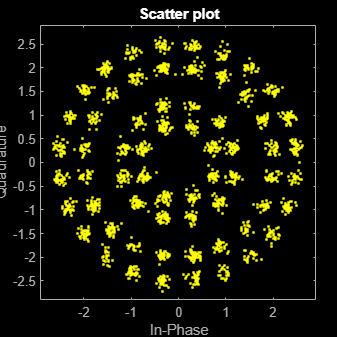## Input Arguments

collapse all

Input signal, specified as a scalar, vector, or matrix. The elements of x must be binary values or integers in the range [0, (`sum(M)-1`)].

Note

To process the input signal as binary elements, set the '`InputType`' name-value pair to `'bit'`. For binary inputs, the number of rows must be an integer multiple of log2(sum(M)). Groups of log2(sum(M)) bits in a column are mapped onto a symbol, with the first bit representing the MSB and the last bit representing the LSB.

Data Types: `single` | `double` | `int8` | `int16` | `int32` | `uint8` | `uint16` | `uint32` | `logical`

Constellation points per PSK ring, specified as a vector with more than one element. Each vector element indicates the number of constellation points in its corresponding PSK ring. The first element corresponds to the innermost circle, and so on, until the last element, which corresponds to the outermost circle. Element values must be multiples of four and `sum(M)` must be a power of two. The modulation order is the total number of points in the signal constellation and equals the sum of the vector elements, `sum(M)`.

Example: `[4 12 16]` specifies a three PSK ring constellation with a modulation order of `sum(M)` = 32.

Data Types: `double`

Radius per PSK ring, specified as a vector with the same length as `M`. The first element corresponds to the innermost circle, and so on, until the last element, which corresponds to the outermost circle. The elements must be positive and arranged in increasing order.

Example: `[0.5 1 2]` defines radii for three constellation PSK rings. The inner ring has a radius of 0.5, the second ring has a radius of 1.0, and the outer ring has a radius of 2.0.

Data Types: `double`

Phase offset per PSK ring in radians, specified as a scalar or vector with the same length as `M`. The first element corresponds to the innermost circle, and so on, until the last element, which corresponds to the outermost circle. The `phaseoffset` can be a scalar only if all the elements of `M` are the same value.

Example: `[pi/4 pi/12 pi/16]` defines three constellation PSK ring phase offsets. The inner ring has a phase offset of pi/4, the second ring has a phase offset of pi/12, and the outer ring has a phase offset of pi/16.

Data Types: `double`

### Name-Value Arguments

Specify optional comma-separated pairs of `Name,Value` arguments. `Name` is the argument name and `Value` is the corresponding value. `Name` must appear inside quotes. You can specify several name and value pair arguments in any order as `Name1,Value1,...,NameN,ValueN`.

Example: ```y = apskmod(x,M,radii,'InputType','bit','OutputDataType','single');```

Symbol mapping, specified as the comma-separated pair consisting of `'SymbolMapping'` and one of the following:

• `'contourwise-gray'` — Uses Gray mapping along the contour in the phase dimension for each PSK ring.

• `'gray'` — Uses Gray mapping along the contour in both the amplitude and phase dimensions. For Gray symbol mapping, all the values for `M` must be equal and all the values for `phaseoffset` must be equal. For a description of the Gray mapping used, see .

• integer vector — Use custom symbol mapping. Vector must consist of `sum(M)` unique elements with values in the range [0, (`sum(M)-1`]. The first element corresponds to the constellation point in the first quadrant of the innermost circle, with subsequent elements positioned counterclockwise around the PSK rings.

The default symbol mapping depends on `M` and `phaseOffset`. When all the elements of `M` are equal and all the elements of `phaseOffset` are equal, the default is `'gray'`. For all other cases, the default is `'contourwise-gray'`.

Data Types: `double` | `char` | `string`

Input type, specified as the comma-separated pair consisting of `'InputType'` and either of these options:

• `'integer'` –– The input signal must consist of integers in the range [0, (`sum(M)` – 1)].

• `'bit'` –– The input signal must contain binary values, and the number of rows must be an integer multiple of log2(sum(M)). Binary input signals are assumed to be left-MSB aligned and specified column-wise. Groups of log2(sum(M)) bits in a column are mapped onto a symbol, with the first bit representing the MSB and the last bit representing the LSB.

Data Types: `char` | `string`

Output data type, specified as the comma-separated pair consisting of `'OutputDataType'` and either `'double'` or `'single'`.

Data Types: `char` | `string`

Plot reference constellation, specified as the comma-separated pair consisting of `'PlotConstellation'` and a logical scalar. To plot the reference constellation, set `PlotConstellation` to `true`.

Data Types: `logical`

## Output Arguments

collapse all

APSK modulated signal, returned as a complex scalar, vector, or matrix. The dimensions of `y` depend on the specified `'InputType'` value.

`InputType`Dimensions of `y`
`'integer'``y` has the same dimensions as input `x`.
`'bit'`The number of rows in `y` equals the number of rows in `x` divided by log2(sum(`M`)).

## Algorithms

The function implements a pure APSK constellation.

A pure M-APSK constellation is composed of NC concentric rings or contours, each with uniformly spaced PSK points. The M-APSK constellation set is

$\chi =\left\{\begin{array}{cc}{R}_{1}\mathrm{exp}\left(j\left(\frac{2\pi }{{M}_{1}}i+{\theta }_{1}\right)\right),& i=0,\dots ,{M}_{1}-1,\\ {R}_{2}\mathrm{exp}\left(j\left(\frac{2\pi }{{M}_{2}}i+{\theta }_{2}\right)\right),& i=0,\dots ,{M}_{2}-1,\\ ⋮& ⋮\\ {R}_{{N}_{\text{C}}}\mathrm{exp}\left(j\left(\frac{2\pi }{{M}_{{N}_{\text{C}}}}i+{\theta }_{\text{Nc}}\right)\right),& i=0,\dots ,{M}_{{N}_{\text{C}}}-1,\end{array}$

where

• The modulation order is equal to the sum of all Ml for l = 1, 2, ... , NC.

• NC is the number of concentric rings. NC ≥ 2.

• Ml is the number of constellation points in the lth ring.

• Rl is the radius of the lth ring.

• θl is the phase offset of the lth ring.

• $j=\sqrt{-1}$

 Corazza, Giovanni E. Digital Satellite Communications. New York: Springer Science Business Media, LLC, 2007.

 Liu, Z., Q. Xie, K. Peng, and Z. Yang. "APSK Constellation with Gray Mapping." IEEE Communications Letters. Vol. 15, Number 12, December 2011, pp. 1271–1273.